### Home > CAAC > Chapter 7 > Lesson 7.3.3 > Problem7-109

7-109.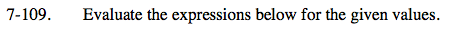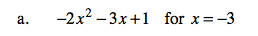Substitute the given value in for the variable and then follow the order of operations to evaluate.

−2(−3)2 − 3(−3) + 1
−2(9) − (−9) + 1
−18 +10
−8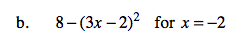Follow the steps shown in part (a) to solve part (b).

−56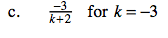Use the same strategy as part (a) to solve part (c).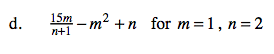See the method used in part (a) for help solving part (d). Remember to substitute for both variables.